CBSE Class 12 Sample Paper for 2019 Boards

Class 12
Solutions of Sample Papers and Past Year Papers - for Class 12 Boards

Question 28

A manufacturer makes two types of toys A and B. Three machine are needed for this purpose and the time (in minutes) required for each toy on the machines is given below:

 Types of Toys Machines I II III A 20 10 10 B 10 20 30

The machines I, II and III are available for a maximum of 3 hours, 2 hours and 2 hours 30 minutes respectively. The profit on each toy of type A is Rs 50 and that of type B is Rs 60. Formulate the above problem as a L.P.P and solve it graphically to maximize profit.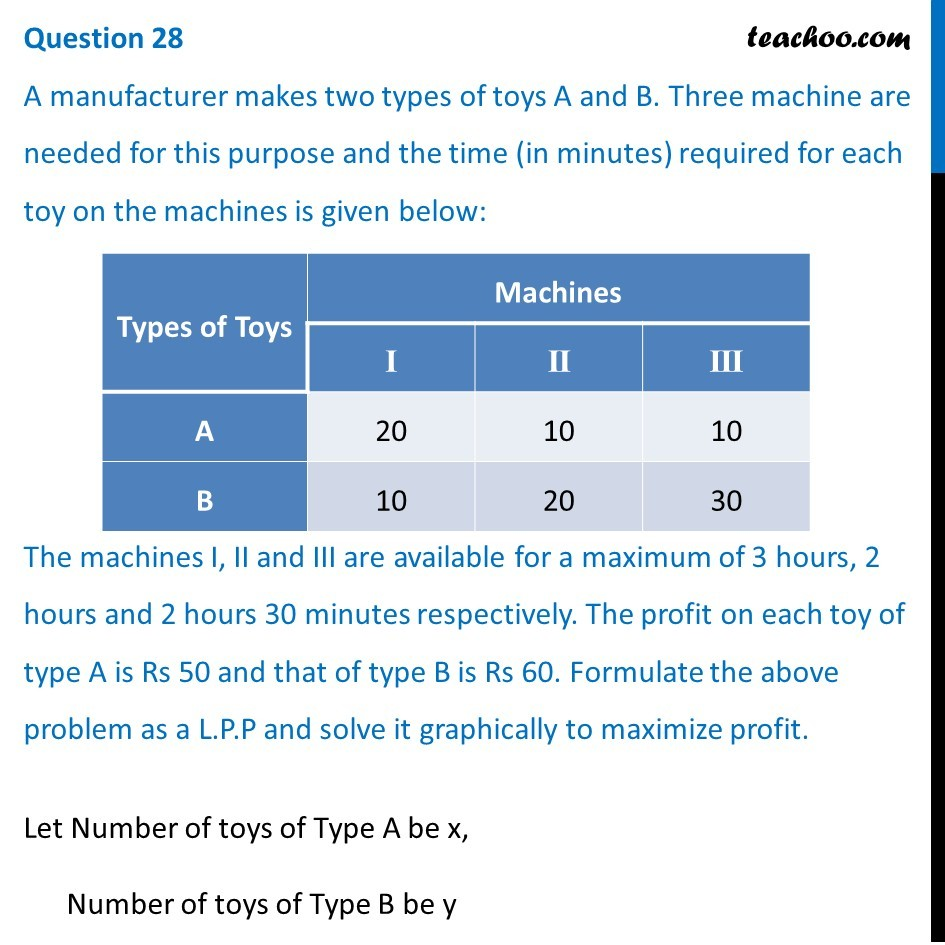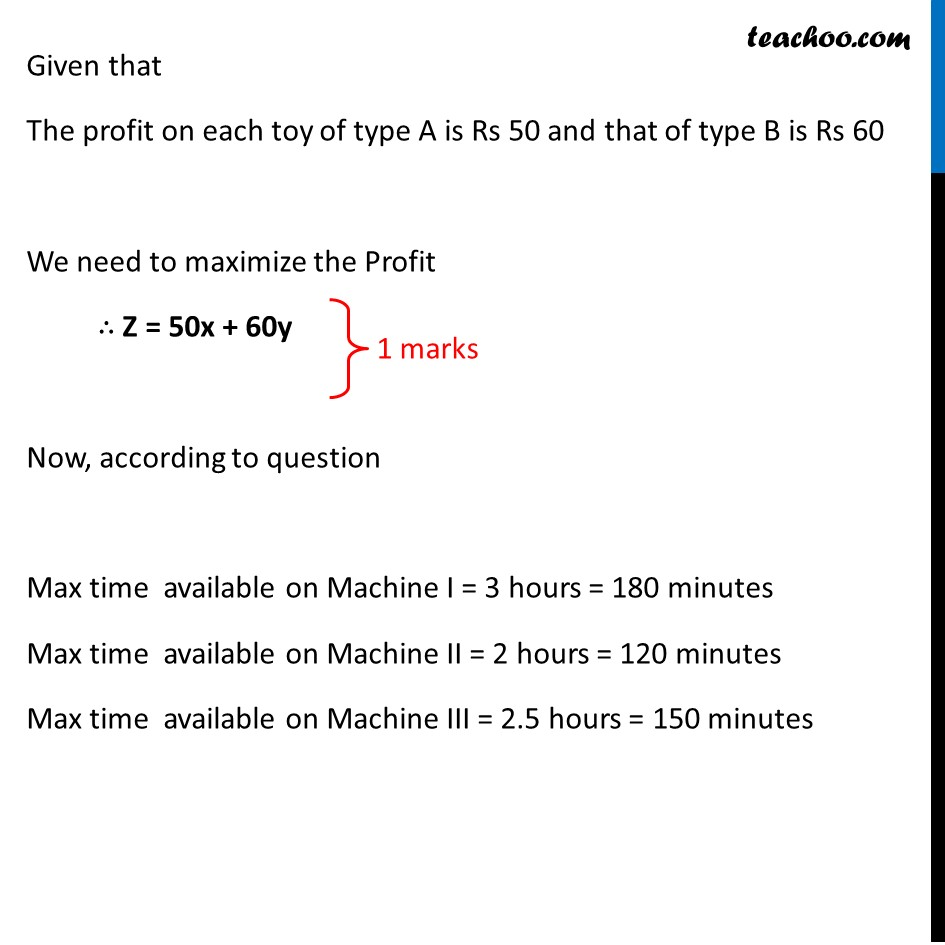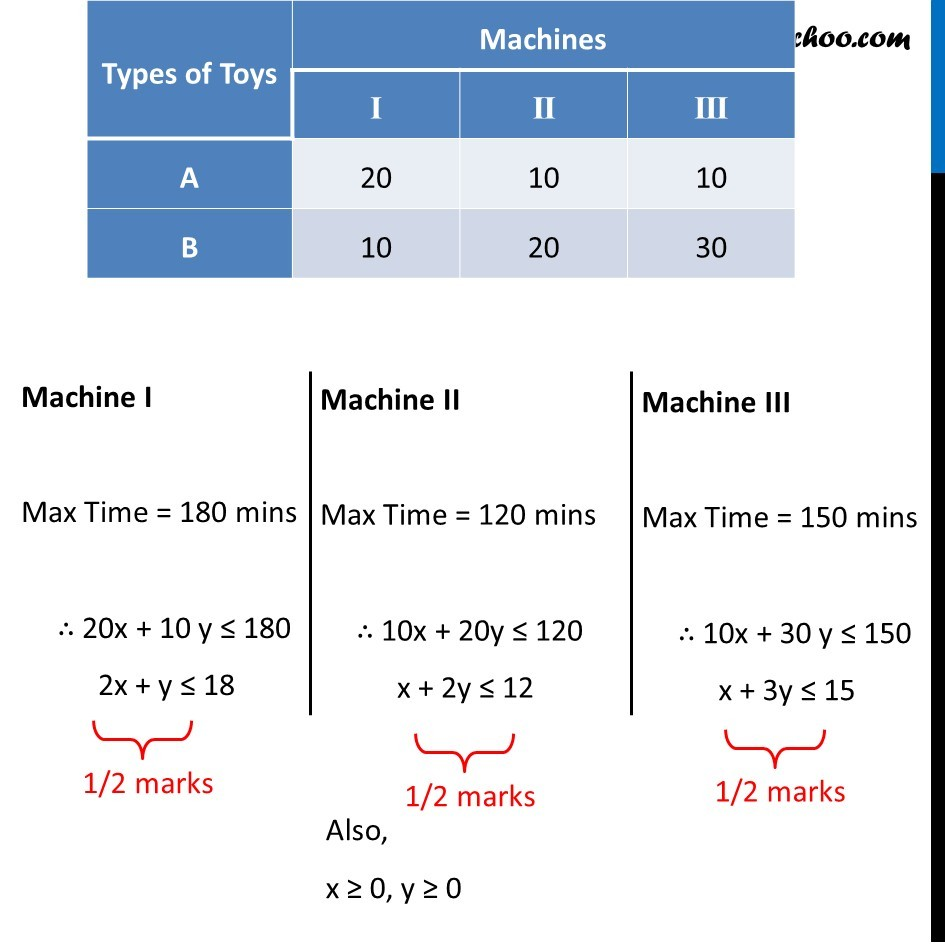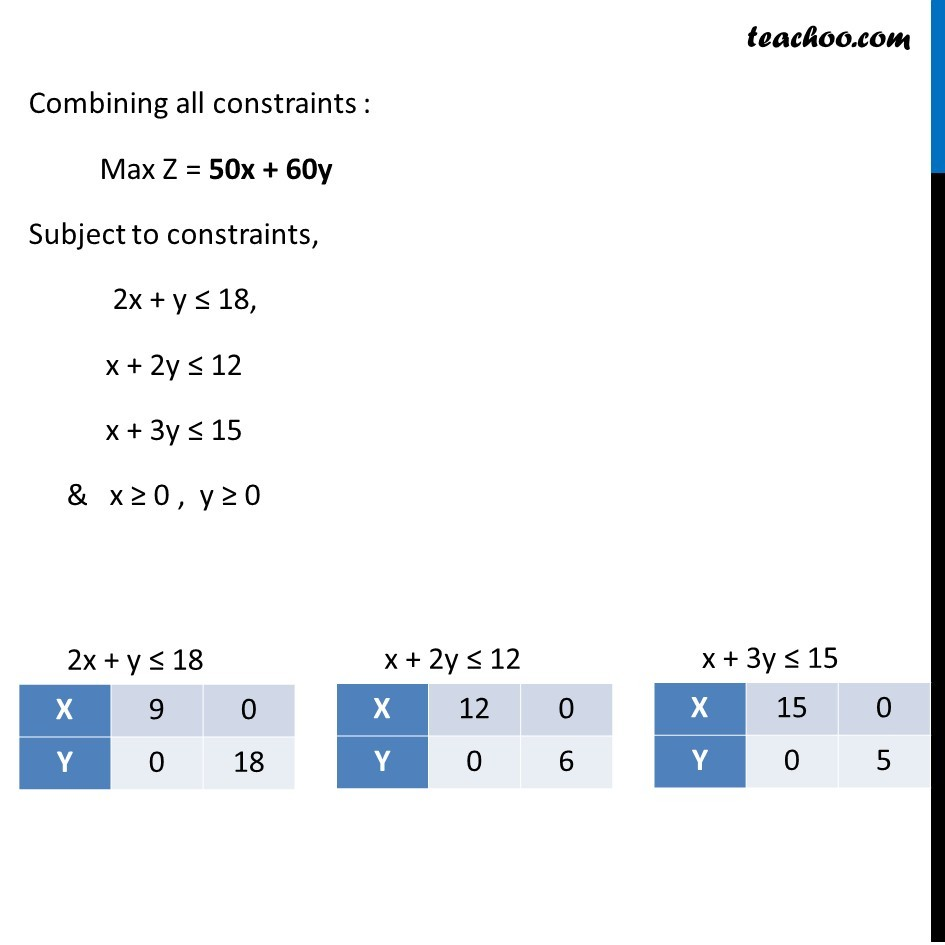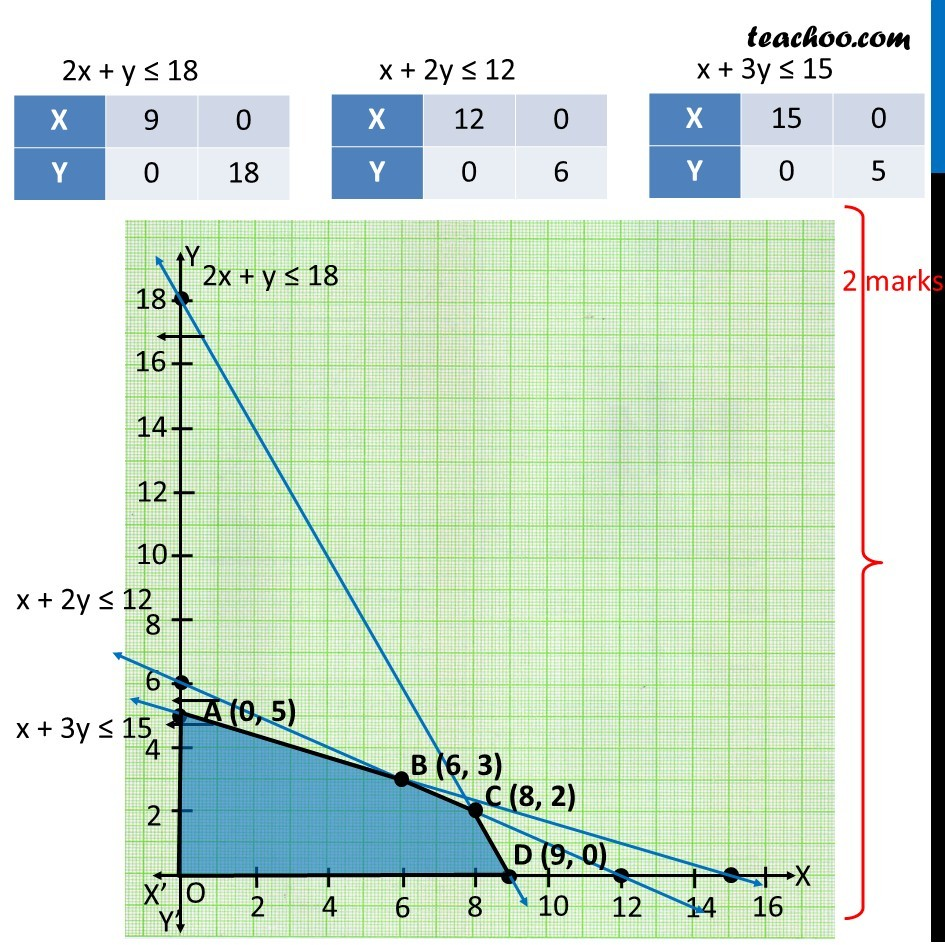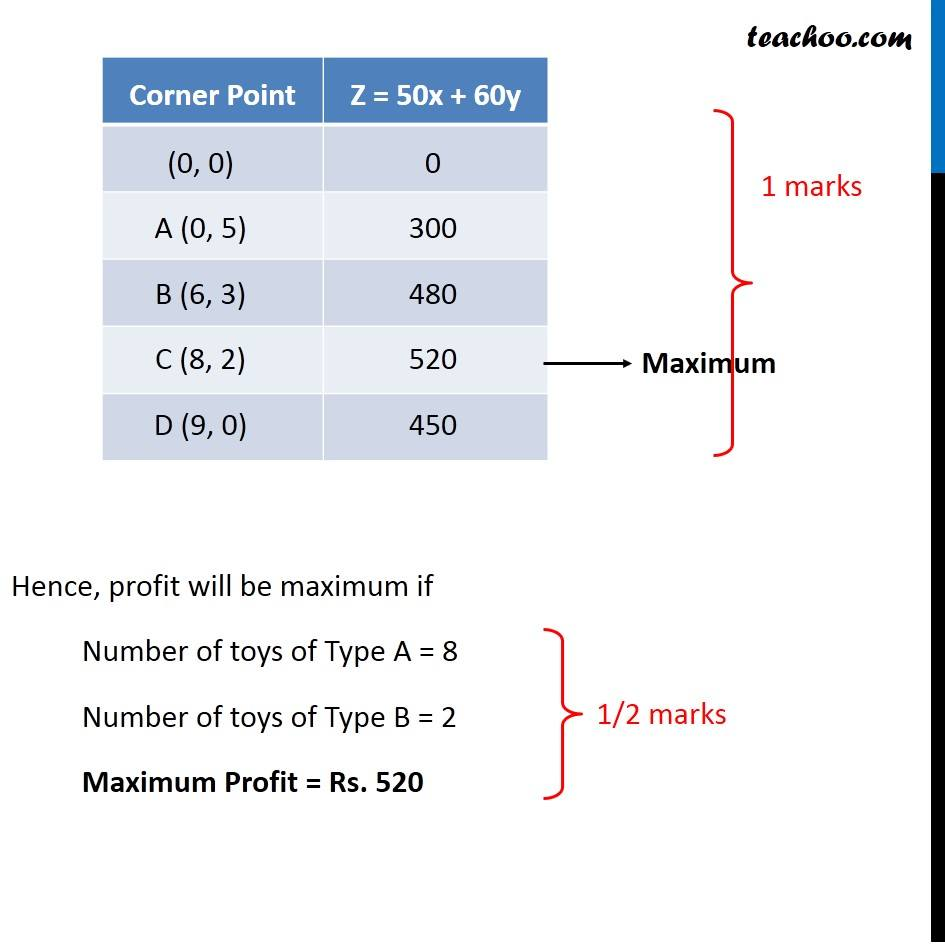Learn in your speed, with individual attention - Teachoo Maths 1-on-1 Class

### Transcript

Question 28 A manufacturer makes two types of toys A and B. Three machine are needed for this purpose and the time (in minutes) required for each toy on the machines is given below: The machines I, II and III are available for a maximum of 3 hours, 2 hours and 2 hours 30 minutes respectively. The profit on each toy of type A is Rs 50 and that of type B is Rs 60. Formulate the above problem as a L.P.P and solve it graphically to maximize profit. Let Number of toys of Type A be x, Number of toys of Type B be y Given that The profit on each toy of type A is Rs 50 and that of type B is Rs 60 We need to maximize the Profit ∴ Z = 50x + 60y Now, according to question Max time available on Machine I = 3 hours = 180 minutes Max time available on Machine II = 2 hours = 120 minutes Max time available on Machine III = 2.5 hours = 150 minutes Machine I Max Time = 180 mins ∴ 20x + 10 y ≤ 180 2x + y ≤ 18 Machine II Max Time = 120 mins ∴ 10x + 20y ≤ 120 x + 2y ≤ 12 Machine III Max Time = 150 mins ∴ 10x + 30 y ≤ 150 x + 3y ≤ 15 Machine III Max Time = 150 mins ∴ 10x + 30 y ≤ 150 x + 3y ≤ 15 Combining all constraints : Max Z = 50x + 60y Subject to constraints, 2x + y ≤ 18, x + 2y ≤ 12 x + 3y ≤ 15 & x ≥ 0 , y ≥ 0 2x + y ≤ 18 X 9 0 Y 0 18 x + 2y ≤ 12 X 12 0 Y 0 6 x + 3y ≤ 15 X 15 0 Y 0 5 Corner Point Z = 50x + 60y (0, 0) 0 A (0, 5) 300 B (6, 3) 480 C (8, 2) 520 -> Maximum D (9, 0) 450 Hence, profit will be maximum if Number of toys of Type A = 8 Number of toys of Type B = 2 Maximum Profit = Rs. 520